#1
Software Development

## The gentlest introduction to the Programming Pattern known as “monads”

PicCollage 的 Design Patterns 系列文章到一個尾聲了。最後一篇向 Jaime（我們的 CTO）邀稿來解釋什麼是 monads! 下台一鞠躬！## Objective

We will learn the programming pattern referred to as a “monad”, but without any of the theoretical rigamarole and a (relatively) practical example, using normal (non-Functional Programming) constructs.

## Why?

The concept of a “monad” is one of the more confusing/unclear ideas developers run into while learning Functional Programming (FP) techniques. Even when not developing full on FP, it is still very useful as a pattern, as evidenced by its use in the Rx framework, Arrays/Iterables and jQuery.

There is no shortage explanations written about “monads””, but I thought it would be useful to understand (1) why it is useful as a design pattern (2) how to use it, by building up the pattern from scratch.

## The Setup

For these examples we are using TypeScript (a typed superset of JavaScript) which is generic enough that it should be understandable by anyone who’s used a modern programming/scripting language.

Suppose our application requires 3 entities:

``````class Db {
constructor(readonly id: string = "") {}
}
export class User {
constructor(readonly name: string = "") {}
}
export class Collage {
constructor(readonly title: string = "") {}
}
``````

In TypeScript this defines 3 classes with one data property in each.

Suppose further that have 3 procedures/functions for loading these objects, dependent on each other, that in the end we have to connect together:

``````getDb() {
// Do some stuff
return new Db();
}
function getUser(db: Db) {
// Do some stuff from Db to get User
return new User();
}
function getCollage(user: User) {
// Do some stuff from User to get Collage
return new Collage();
}
``````

Our ultimate goal is to “load a Collage” and for that we need to get a `Db`, use it to get a `User`, and then use that to get a `Collage`, thusly:

``````loadCollage() {
const db      = getDb();
const user    = getUser(db);
const collage = getCollage(user);
return collage;
};
``````

We also could have written loadCollage with less temporary variables, like this:

``````function loadCollage1() {
return getCollage(
getUser(
getDb()
)
)
}
``````

But that is hard to read because it is written “backwards”, forcing us to write `getCollage()` first, and `getDb()` last even though `getDb()` is executed first.

As a bit of syntactic sugar, imagine if our objects had a simple method which when given an anonymous function `f` (called a lambda), simply applies that function `f` to itself and returns what that function returns.

Bear with us, we will see in a moment, why this would be useful.

Let’s call that method `map`, because such a method would take an object of one type (e.g. class Db) and “map” it to an object of another class (e.g. class User).
Thus on the `Db` class it would be this:

``````class Db {
...
map<R>(f: (_this: Db) => R) {
return f(this)
}
}
``````

Our method `map` simply takes a function `f`, runs the function with itself as an argument and returns.

It is not necessary to understand the details of the TypeScript, but for the curious, the “type” of `f` is `(db: Db) => R` which means "anonymous function that takes a `Db` and returns an `R`". The whole method is "generic" on "R" because "R" can be whatever type is returned from the given function.

Thus the code to get a `User` from a `Db` can be written like this:

``````db   = getDb()
const user = db.map(_db => getUser(_db))
``````

We can replace the lambda `db => getUser(db)` with just simply a reference to that function `getUser`:

``````db   = getDb()
const user = db.map(getUser)
``````

or

``````user = getDb().map(getUser)
``````

and ultimately, if all classes had a `map` method, we can write our final method like this:

``````function loadCollage() {
return getDb()
.map(getUser)
.map(getCollage);
};
``````

And this is very readable! we are just saying “get a Db, map that to a User, then map that to a Collage”. Very concise, no noise from temporary variables, etc. and it fixes the “backwards” readability problem.

## Yay!

Take a break and appreciate that we are now doing Functional Programming, applying functions like a pro. There are no “imperative” steps or statements in this version of `loadCollage`.

## Wait, what if there’s a problem?

Imagine that sometimes the database is not available, so `getDb()` fails. In this case let’s say `getDb()` returns null. How does our code change? we'd have to either check before each call:

``````function loadCollageImperative() {
const db      = getDb();
const user    = db ? getUser(db) : null;
const collage = user ? getCollage(user) : null;
return collage;
};
``````

which is harder to read, or we could change `getUser` and `getCollage` to accept `null` failed arguments and pass on the failure by returning `null` also:

``````function getUser(db: Db|null) {
if (db === null)
return null
return new User();
}
``````

Neither of these two options is great from a separation of concerns perspective: We want the `getUser` function to be concerned with getting the User, not dealing with the previous failure of the database. We want `loadCollage` to not be burdened with error checking every step of the way.

## Pipe dreams

Let’s consider what our functions do: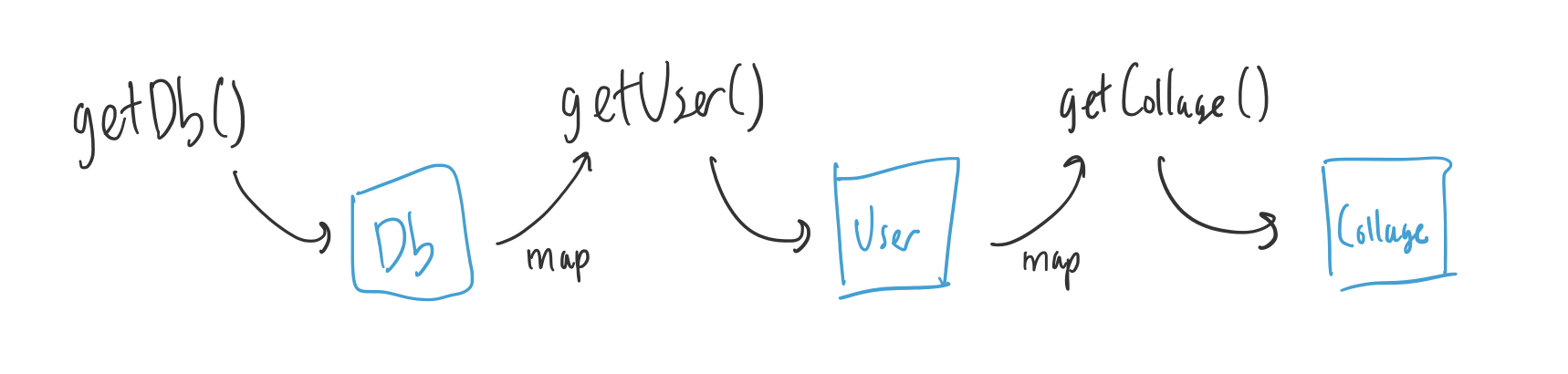The `getDb` produces a `Db` object, which then the function `getUser` "converts" to a `User`, and which then the function `getCollage` "converts" to a `Collage`. Remember that we are using `map` to apply those `getUser` and `getCollage` functions. You can think of these functions as “pipes” that change (“map”) one type of object to another.

Imagine that we had this “box” (or wrapper) that could either contain another object or be empty (represented by a `null`).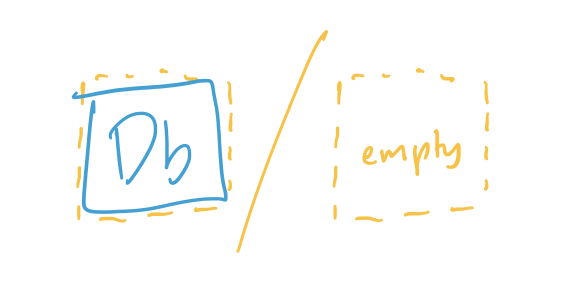More importantly this box would support the `map` method for applying functions. `map` in this box would work the same as the normal `map`, except it would:

1. Take the value object out of the box.
2. Apply the function to the value object to get a result.
3. Repackage the result back into the same kind of box and return that.

We can call this box `Maybe` since it “maybe contains a value”:

``````class Maybe<T> {

map<R>(f: (t: T) => R): Maybe<R> {
if (this.value === null)
return new Maybe<R>(null);
return new Maybe(f(this.value));
}

}
``````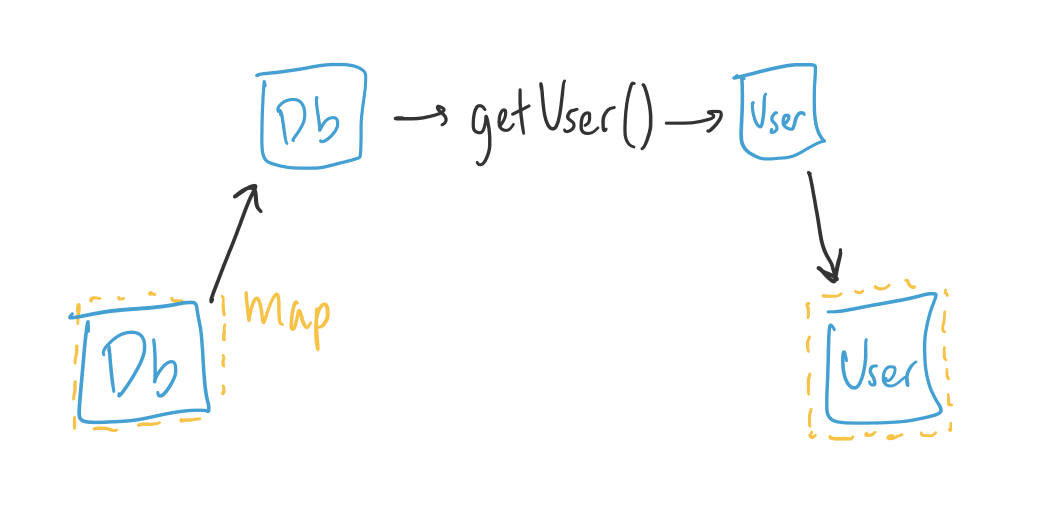Because we are using `map` to apply the functions, the functions do not have to know anything about boxes or the possibility the data and can stay exactly the same and `loadCollage` is mostly the same.

``````function loadCollage() {
return new Maybe(getDb())
.map(getUser)
.map(getCollage)
};
``````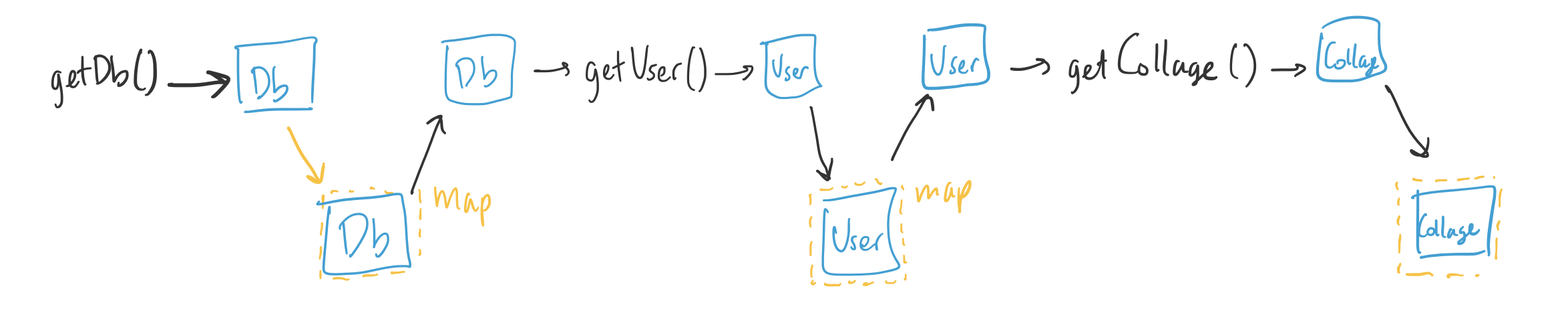And so this is great because we have separated the concerns and functionality between (1) getting `Db`, `User` and `Collage` (thru the functions `getDb`, `getUser`, `getCollage`), and (2) dealing with the failure to load (which is all dealt with in the `Maybe` box class).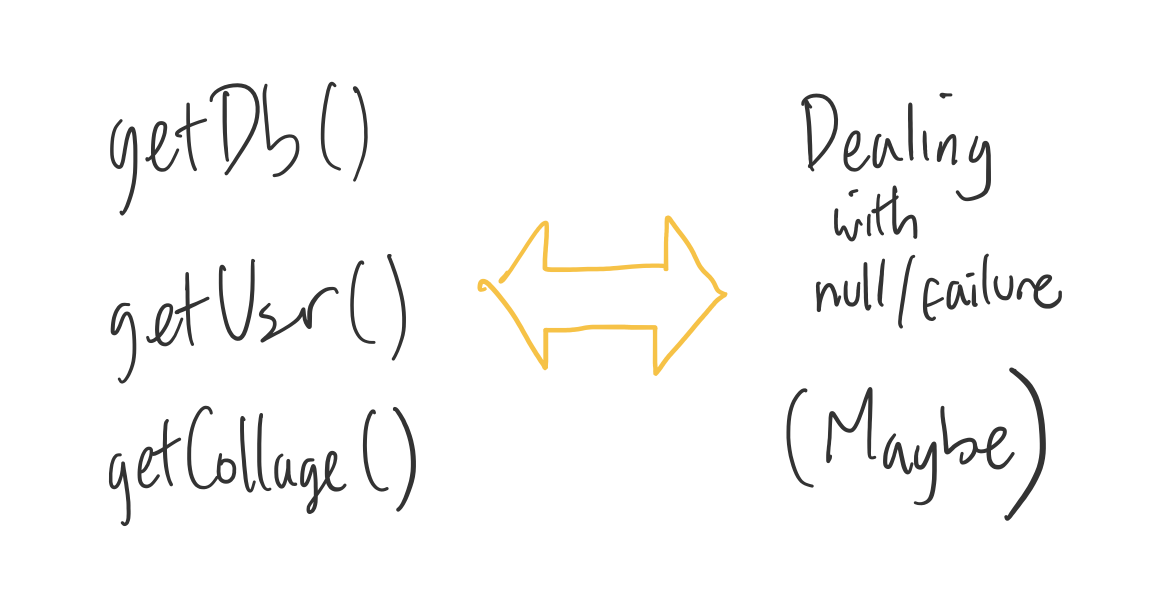And so the pattern of separating functionality here can be extended to other things, not just dealing with null/failure. For example it can be “dealing with many of the same thing” (i.e. `Array`, which in most languages supports `map`), or dealing with objects arriving over time (i.e. `Observable` from the Rx framework). Other examples are query objects in jQuery and maybe query objects in Ruby-on-Rails/ActiveRecord.

For example, `map` on `Array` lets us operate on objects in an `Array` or an `Iterable`, one by one, without knowing about the Array itself or Iteration.

``````const a = [1,2,3,4]
const f = (n) => n * 2    // Function just knows about multplying by 2
a.map(f)                  // This will return [2,4,6,8]
``````

`Observables`, which are values over time, also implement `map`:

``````const obs = rxmarbles('----1---2-----3-----4----|')
const f1 = (n) => n * 10   // Function just knows about multplying by 10
const f2 = (n) => n + 1    // Function just knows about adding 1
a.map(f1)                  // This will return rxmarbles('----10---20-----30-----40----|')
a.map(f1)
.map(f2)                  // This will return rxmarbles('----11---21-----31-----41----|')
``````

The boxes we just finished describing are called ‘functors’. The main requirement for a functor is to be a “box” (a container for another type/class) and support map for applying functions to its contents.

Up to now, the functions we’ve been applying have remained blissfully unaware and separated from the concerns of the “box”/functor. For example, `getUser()` doesn't deal or produce anything related to the `null` value, and this is a good thing.

However, there are some situations in which the function does need to produce something related to the concerns of the “box”. For example, maybe `getUser()` could also sometimes fail and wants to return `null`. So we could redefine `getUser()` to return the `Maybe` functor to signal that it failed, like:

``````function getUser(db: Db): Maybe<User> {
if (\$SOMEERROR)
return new Maybe(null);
else
return new Maybe(new User());
}
``````

And we could also do the same with `getCollage()`. However, if we stuck to our original code of:

``````function loadCollage() {
return getDb()
.map(getUser)
.map(getCollage)
};
``````

we would have a problem because, remember, `map` takes the contents of the box, applies the function, and then puts the results inside a new box. So we would end up with a double box wrapping!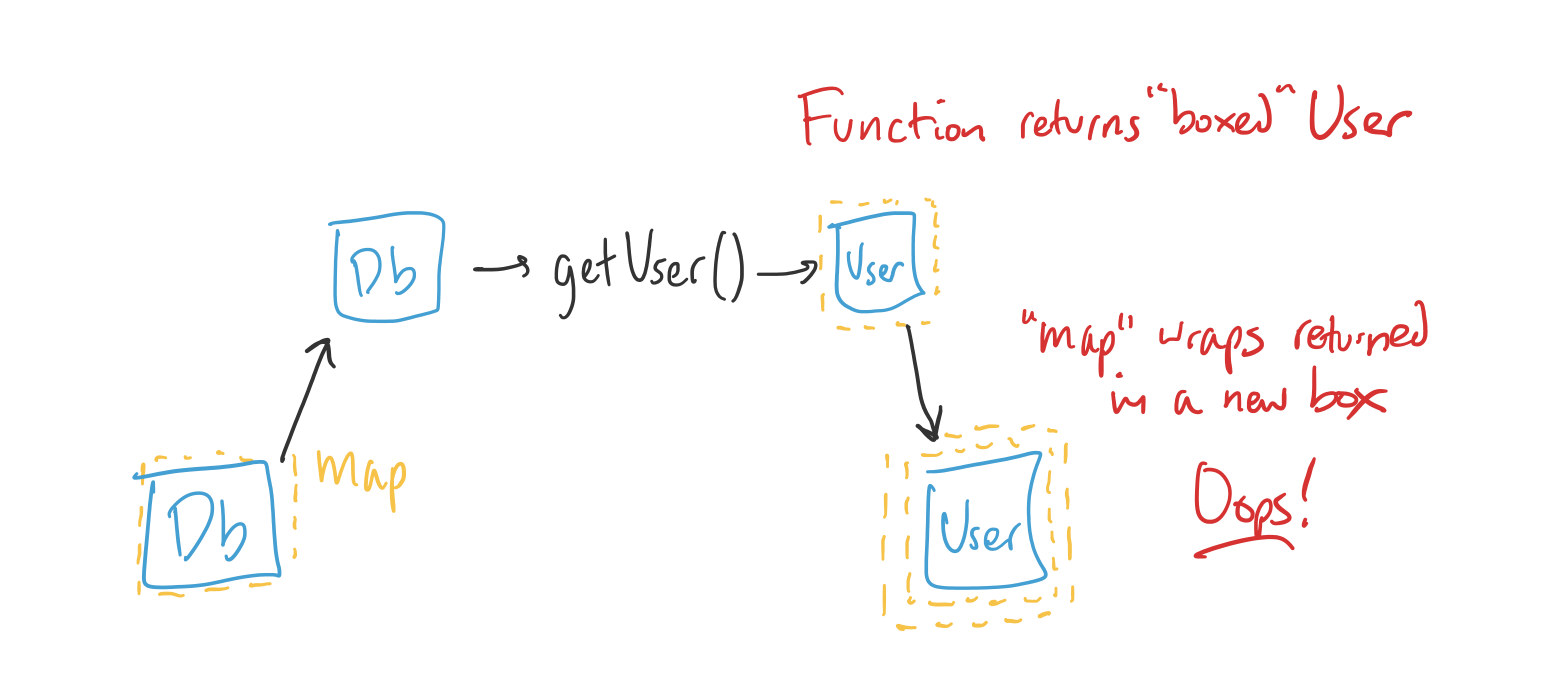So what we need is a different kind of `map` that expects the functions to return an already boxed value, deal with it somehow, and then return a singly boxed value.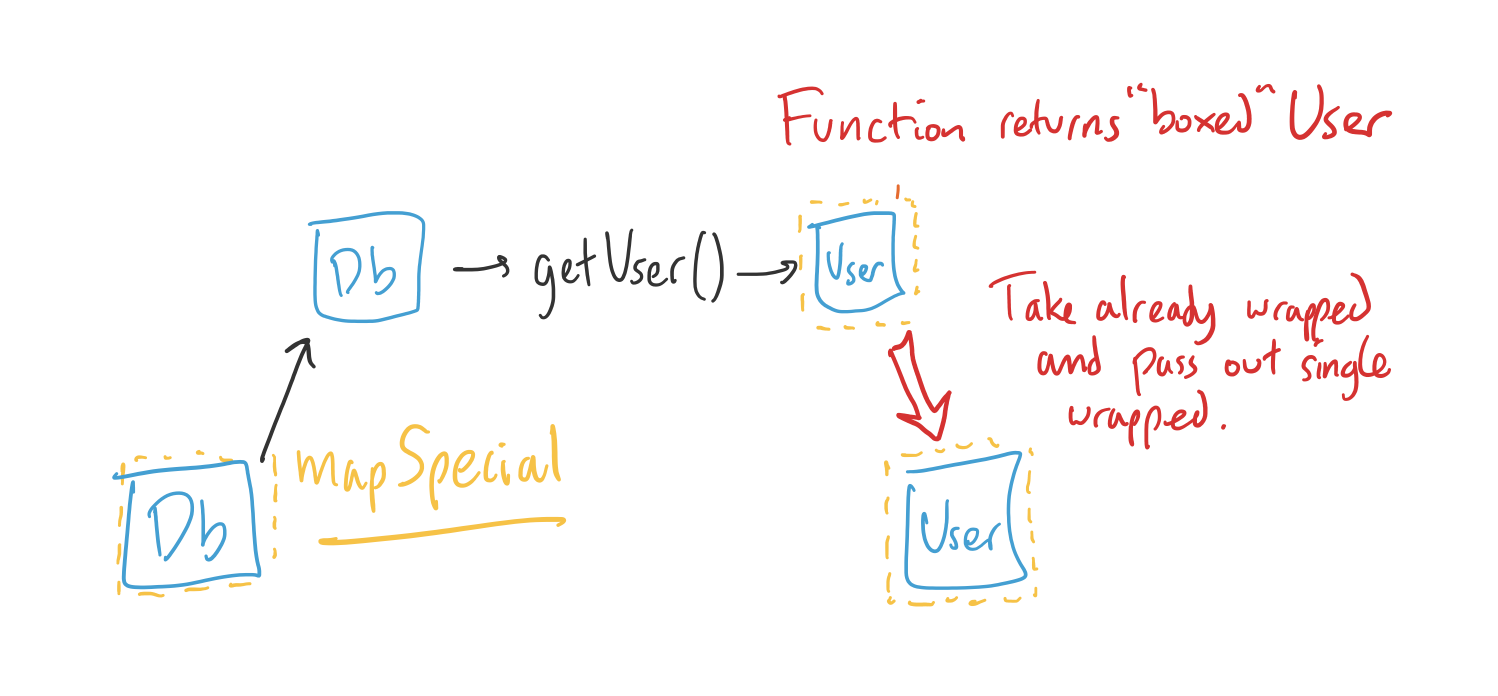The simplest case is one in which it just returns the same box:

``````// ---- Map that just flattens
map2<R>(f: (t: T) => R): Maybe<R> {
if (this.value === null)
return new Maybe<R>(null);
// return new Maybe(f(this.value));     // No need to re-box!
return f(this.value);
``````

This is usually called the “flatMap” because it “flattens” out the boxes into just one.

``````function loadCollage() {
return getDb()
.flatMap(getUser)
.flatMap(getCollage)
};
``````

But you could also have a different `map` that, for example, retries until the function succeeds:

``````// ---- Map that keeps retrying
mapRetry<R>(f: (t: T) => R): Maybe<R> {
if (this.value === null)
return new Maybe<R>(null);
while (1) {
const ret = f(this.value);
if (ret.value)
return ret;     // Succeeded!
// Wait a bit and loop to try again
sleep(1000);
}
``````

And this, in a nutshell is what a “monad” is: when the “box” supports

1. The normal `map` that works with functions that return unboxed values, like a “functor”.
2. At least one special method like `flatMap` that works with these functions that return already boxed values.

In the case of the `Array` monad for example, the "box" is the Array, so `flatMap` works like this:

``````a = [1, 2, 3]
const f = i => [i, i, i]   // Function gets a value and returns a triplicated Array
a.flatMap(f)               // This returns [1,1,1,2,2,2,3,3,3],
// because `flatMap` concatenates all the Arrays
// the function returns.
``````

In the case of the `Observable` monad for example, the "box" is a series of values over time, and because there's different ways to combine the result of function there's many different kinds of "maps":

``````a = rxmarbles('---1----2--------3-------|)
const f = v => rxmarbles('---v--v--v-!')
a.mergeMap(f)
// This takes all the outputs and just overlaps them.
// Returns rxmarbles('---1--1-21-2--2--3--3--3------|')
a.concatMap(f)
// This takes all the outputs and puts them one after the other.
// Returns rxmarbles('---1--1--1-2--2--2---3--3--3----|')
a.switchMap(f)
// This only outputs the latest series and stops the previous one.
// Returns rxmarbles('---1--1-2--2--2--3--3--3------|')
``````

## Conclusion

To summarize, there is nothing magical about “functors” and “monads”. We can think about them as just a programming pattern that lets us separate the different concerns and functionality of our data. For example, separate the intrinsic app-specific properties, from the Maybe-ness, from the Array-ness, from Observable-ness, etc., and apply them as separately composeable functions. Thanks for reading and let me know of any questions in the comments!

Author: Jaime